Next: Partial Waves Up: Scattering Theory Previous: Fundamentals

# Born Approximation

Equation (1266) is not particularly useful, as it stands, because the quantity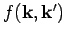depends on the, as yet, unknown wavefunction[see Eq. (1261)]. Suppose, however, that the scattering is not particularly strong. In this case, it is reasonable to suppose that the total wavefunction,, does not differ substantially from the incident wavefunction,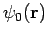. Thus, we can obtain an expression forby making the substitution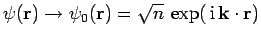in Eq. (1261). This procedure is called the Born approximation.

The Born approximation yields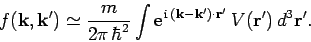(1267)

Thus,is proportional to the Fourier transform of the scattering potentialwith respect to the wavevector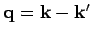.

For a spherically symmetric potential,(1268)

giving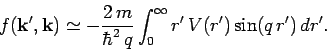(1269)

Note that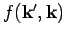is just a function of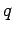for a spherically symmetric potential. It is easily demonstrated that(1270)

whereis the angle subtended between the vectorsand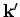. In other words,is the scattering angle. Recall that the vectorsandhave the same length, via energy conservation.

Consider scattering by a Yukawa potential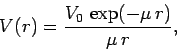(1271)

where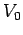is a constant, andmeasures the range'' of the potential. It follows from Eq. (1269) that(1272)

since(1273)

Thus, in the Born approximation, the differential cross-section for scattering by a Yukawa potential is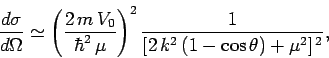(1274)

given that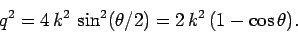(1275)

The Yukawa potential reduces to the familiar Coulomb potential as, provided that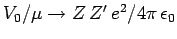. In this limit, the Born differential cross-section becomes(1276)

Recall thatis equivalent to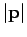, so the above equation can be rewritten(1277)

whereis the kinetic energy of the incident particles. Of course, Eq. (1277) is the famous Rutherford scattering cross-section formula.

The Born approximation is valid provided thatis not too different fromin the scattering region. It follows, from Eq. (1258), that the condition for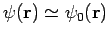in the vicinity of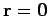is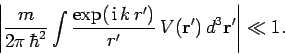(1278)

Consider the special case of the Yukawa potential. At low energies, (i.e.,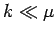) we can replace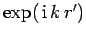by unity, giving(1279)

as the condition for the validity of the Born approximation. The condition for the Yukawa potential to develop a bound state is(1280)

whereis negative. Thus, if the potential is strong enough to form a bound state then the Born approximation is likely to break down. In the high-limit, Eq. (1278) yields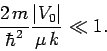(1281)

This inequality becomes progressively easier to satisfy asincreases, implying that the Born approximation is more accurate at high incident particle energies.Next: Partial Waves Up: Scattering Theory Previous: Fundamentals
Richard Fitzpatrick 2010-07-20